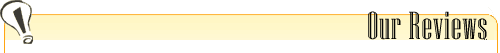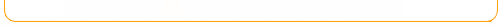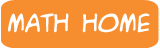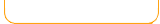Decimal Math Games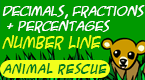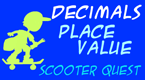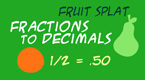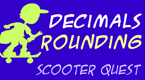Keep checking back for more games!Popular Math Games! Skill Builders! 1)Fractions Splat 1) Fruit Splat Skill Builders - Addition 2) Clock Splat 2) Fruit Splat Skill Builders - Subtraction 3) Number Words 3) Fruit Splat Skill Buiders - Multiples 4) Fruit Splat - Addition 4) Catch the Stars - Addition 5) Fruit Splat - Subtraction 5) Catch the Stars - Multiples 6) Fractions - Addition 6) Croc Doc - Division 7) Fruit Splat Multiplication 7) Decimals - Rounding - Scooter Quest 8) Shapes Splat 8) Mixed Operations - Fruit Splat 9) Odd-Even Fruit Splat 9) Fractions to Decimals - Fruit Splat 10) Subtraction Number Lines 10) Pearl Search - Subtraction 11) Geometry - Line Game 11) Integers - Addition - Fruit Splat 12) Coins Splat - Money Game 12) Greatest Common Factor - Fruit Splat 13) Penguin Party Addition Game 13) Coins 2 -Money Game 14) Scooter Quest - Place Value 14) Group Counting - Fruit Splat 15) Multiplication Mayhem 15) Decimals Place Value - Scooter Quest

We are working on converting our games! Please play the above games that are
more and more of our excellent math games!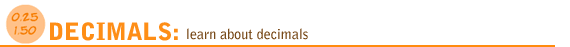Relate Fractions & Decimals Comparing
& Ordering
Decimals
Rounding Patterns Comparing
Fractions &
Decimals

Decimal Models - Tenths and Hundredths
Use models to understand tenths.
Play the Decimal Models Tenths game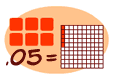Use models to understand hundredths.
Play the Decimal Models Hundredths game to

Relate Fractions and Decimals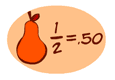Tenths show 10 equal parts. One tenth is shown as 1/10
as a fraction and as 0.1 as a decimal. Play the Fractions to Decimals game to relate fractions and decimals.
Fractions to Decimals 3rd grade and up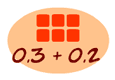What is the sum of 0.1 and 0.2?What is the difference of 0.2 and 0.1?

Comparing and Ordering Decimals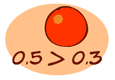Compare decimals.
Is 0.2 greather than 0.1 ?
Yes, two-tenths is greater than one tenth.
Play the Compare Decimals game for more.
Compare Decimals 3rd grade and up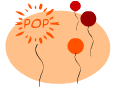Order decimals by popping balloons.
Is 0.1 < 0.2 < 0.3?
Yes. Play the Order Decimals 1 game for more.
For a challenge, play the Order Decimals 2 game.
Order Decimals 1
Order Decimals 2

Decimal Place ValueDoes 0.51 have 5 in the tenth's place?
Place Value 4th grade and up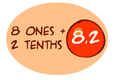Shoot the fruit that has the correct number for the expanded form.
For example, 8 ones and 2 tenths = 8.2
Expanded Form 4th grade and up

Decimal RoundingWhat is 5.6 rounded to the nearest whole number?
Yes, it's 6. Play Decimal Rounding for more practice.

Decimal Patterns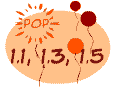In the pattern 0.1, 0.2, 0.3 what decimal comes next?
It's 0.4. For more play Decimal Patterns.

Comparing Decimals and FractionsWhich one is larger, 0.11 or 1/10? Yes, it's 0.11.
play Compare Fractions and Decimals.
Compare Fractions
& Decimals